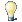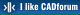#CAD tip # 12777:%  platform  category## Arithmetic and trigonometric functions available in Revit expressions.You can use the following arithmetic, trigonometric and logic functions in entry fields of dimensional values of Revit parametric objects, including RFA families - the expression is always prefixed with the "=" character:

```+ addition
- subtraction
* multiplication
/ division
^ power
() expression separator

ln(expr) - natural logarithm
log(expr) - decimal logarithm
sqrt(expr) - square root
cos(expr) - cosine
sin(expr) - sine
tan(expr) - tangent
acos(expr) - arc cosine
asin(expr) - arc sine
atan(expr) - arc tangent
exp(expr) - e^x
abs(expr) - absolute value
pi - pi (3.1415926536)
round(expr) - rounding to the nearest whole number
rounddown(expr) - nearest lower whole number
roundup(expr) - nearest higher whole number

IF(cond,iftrue,iffalse) - conditional expression
AND(expr) - and
OR(expr) - or
NOT(expr) - negation
< = >  - comparison
example: =IF(OR(A=1,B=3),8,3)
```

You need to keep proper dimensional units in the expression results, e.g. log(100) * 1.0m.

Please note that parameter and object names in Revit are case-sensitive, you need to match lower/UPPER case characters.

 Revit*  CAD 24.10.2020    635×applies to: Revit ·

 See also: Tip 12749: Generate parametric mathematical 3D surfaces (meshes) in Fusion 360. Tip 12638: How to use conditional parameter values in Fusion 360? Tip 12600: Parametric 3D curves in Fusion 360. Tip 11494: Entering decimal values using fractions in CAD applications. Tip 10231: How to draw spiral in AutoCAD LT?

Back   All CAD TipsHave we helped you? If you want to support the CAD Forum web service, consider buying one of our CAD applications, or our custom software development offerings, or donating via PayPal (see above). You may also add a link to your web - like this "fan" link:Featuring:Export 3D VRML scenes from AutoCAD and InventorVRMLout